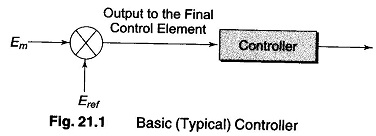## Basic Automatic Control:

Multivariable systems are used more commonly in this age of Basic Automatic Control. Control parameters are becoming more complex by the day and the range of applications wider. In such complex systems, there are more than one input along with noise signals which are also present and there are more than one output. The physical variables such as flow, pressure, temperature, humidity, pH, etc. are to be controlled very closely. These variables may be interde­pendent. In general, the process control systems may he broadly classified as follows :

1. Open loop control systems.
2. Closed loop or feedback control system.
3. Feed forward control system.
5. Ratio control system.

### Basic Control Action

An automatic controller in a process plant works on the principle of a closed loop control system. It compares the actual value of the plant output with the desired or set-point value, a deviation (or error) is obtained which in turn produces a control signal that reduces the error to zero or to an acceptable minimum value. Figure 21.1 shows the diagram of a basic controller.where

e = Error signal to be fed to controller

Em = measured value of the plant variable

Eref = reference or set point value of the variable

The manner in which the control signal (P) produced from the automatic controller, is fed to the final control element is called the control action.

### Error

e=Em-Eref is the expression for error. But, error is most often expressed as a percentage of full scale variable range. It is given by the expression:

where

Ep = error as percentage of range from set-point

Em = measured value of the process variable

Eref = reference, set point of the variable

Emax = maximum value of variable

Emin = minimum value of variable

Emax — Emin = full scale variable range

If Ep is positive, the measured value of the process variable is above the set point and if Ep is negative, it is below the set point value.

In process plant control, the standard range for a process variable is 4-20 mA. If this standard is used, then the current can be substituted to compute the value of deviation.

Scroll to Top